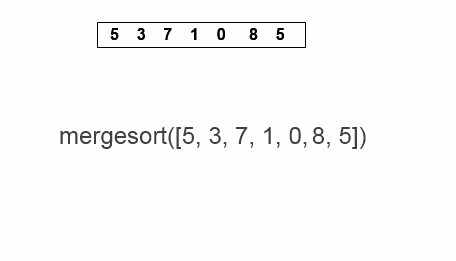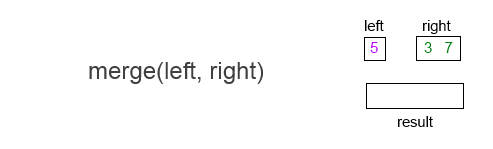Explore BrainMass
Share

# Mergesort

### Implementation

Mergesort (or 'merge sort'), invented in 1945 by John von Neumann, takes a 'divide and conqueror' approach to sorting by splitting an array into individual elements which are trivially sorted and then piecing them back together in ascending order. The array is split up by dividing the length of the array by two (taking the floor of that) and making a sub-array of each part. The reassembling method depends on which first element of each of the two sub-arrays being reassembled is bigger. If the left array's first element is bigger than the right's, they will be reassembled using a merge function but if the right's is bigger, or they are equal, the right array can simply be appended onto the end of the left one.

Here is the pseudocode that specifies this algorithm, taken from Rosetta Code1 (m is the array to be sorted):

function mergesort(m)

var list left, right, result

if length(m) ≤ 1

return m

else

var middle = length(m) / 2

for each x in m up to middle - 1

for each x in m at and after middle

left = mergesort(left)

right = mergesort(right)

if last(left) ≤ first(right)

append right to left

return left

result = merge(left, right)

return result

Below you can see it in action using the example mergesort([5, 3, 7, 1, 0, 8, 5]):The < and > in the gif above mark whether the first element of the left sub-array is less than or greater than that of the right sub-array. Append can be understood immediately but the merge operation is specific to this sorting function. It is done by comparing the first element of each subarray in turn until one is exhausted and the other may be appended on the end. The following is pseudocode for the merge function1:

function merge(left,right)

var list result

while length(left) > 0 and length(right) > 0

if first(left) ≤ first(right)

append first(left) to result

left = rest(left)

else

append first(right) to result

right = rest(right)

if length(left) > 0

append rest(left) to result

if length(right) > 0

append rest(right) to result

return result

See below for an example of this implementation using the first instance described in our example, merging  with [3, 7]:### Performance

Mergesort operates, on average and in worst-case, in O(n log n) time. In addition, it can take advantage of parts of a list/array that are already sorted and so can run faster with these sorts of input. It is also what is known as a stable sort which means the order of elements with the same value is preserved (so in the above example, if some other information were attached to the 5s, they would be in the same order in the output of mergesort as they were going in). This makes it preferable to quicksort in some cases as it performs less comparisons than its fellow O(n log n) sorting algorithm, though it does typically takes more space in memory to perform.

References:

1. Pseudocode from Rosetta Code